# Question 1 2.5 pts A molecule with a square pyramidal molecular geometry has a bond angle...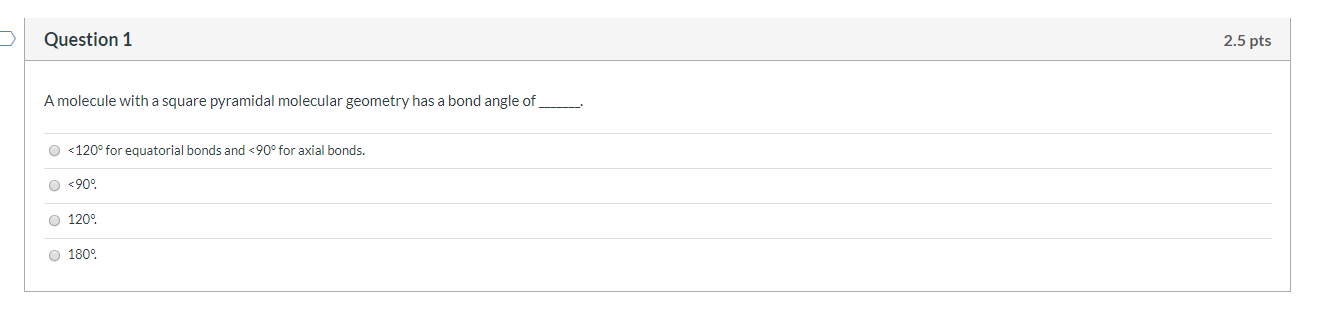Question 1 2.5 pts A molecule with a square pyramidal molecular geometry has a bond angle of <120° for equatorial bonds and <90° for axial bonds. <900 120 180°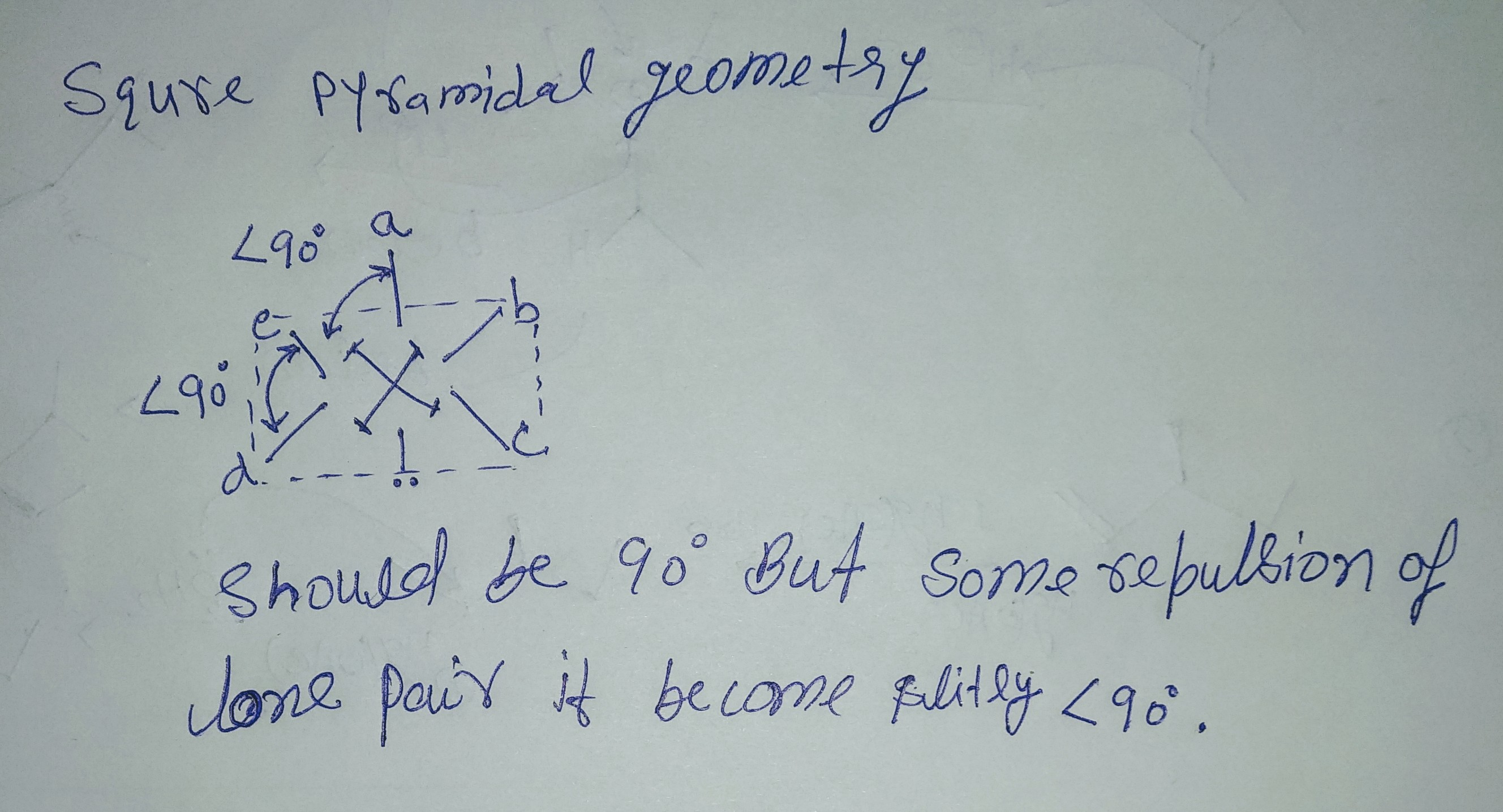#### Earn Coin

Coins can be redeemed for fabulous gifts.

Similar Homework Help Questions
• ### Question 2 2.5 pts A molecule with a seesaw molecular geometry has a bond angle of...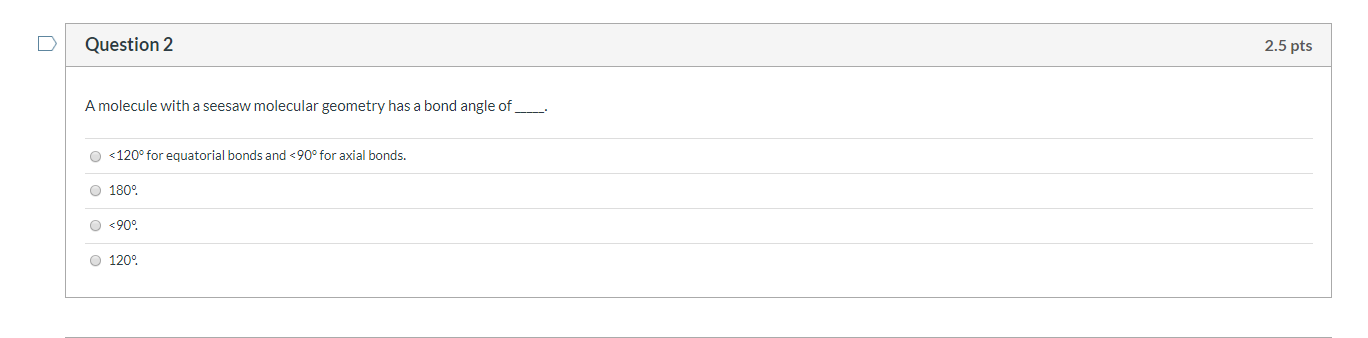Question 2 2.5 pts A molecule with a seesaw molecular geometry has a bond angle of __ <120° for equatorial bonds and <90° for axial bonds. 180° <909 1200

• ### 7- The molecule of NH, is a) tetrahedral with bond angle 109.5° b) pyramidal with bond...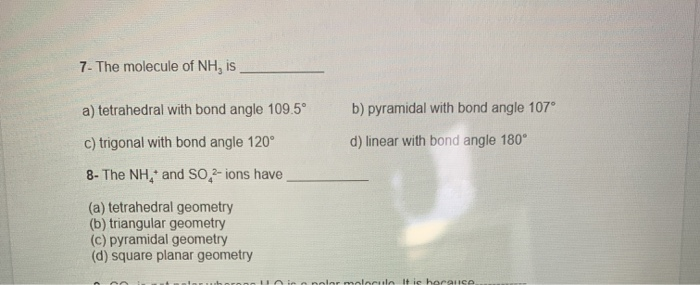7- The molecule of NH, is a) tetrahedral with bond angle 109.5° b) pyramidal with bond angle 107° d) linear with bond angle 180° C) trigonal with bond angle 120° 8- The NH,* and SO2-ions have (a) tetrahedral geometry (b) triangular geometry (c) pyramidal geometry (d) square planar geometry bolor molecule It is horance

• ### Question 10 1 pts Consider the Lewis structure of Br4 , what is the bond angle...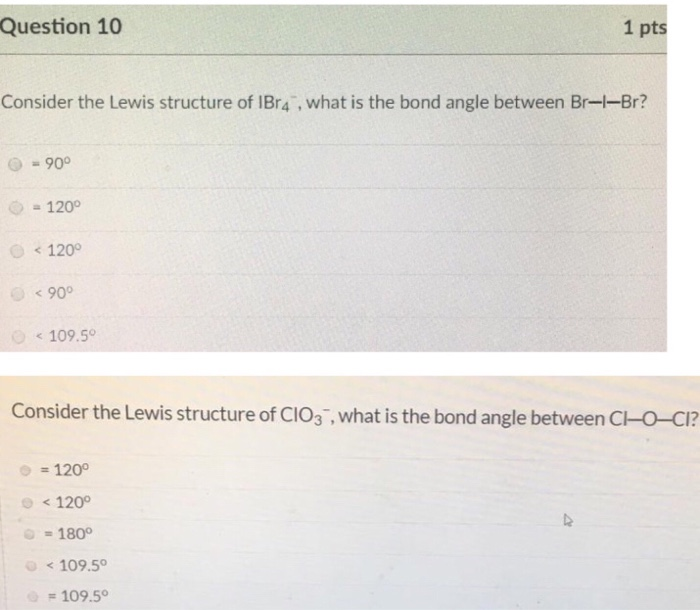Question 10 1 pts Consider the Lewis structure of Br4 , what is the bond angle between Br-1-Br? = 90° = 120° < 120° < 90° < 109.50 Consider the Lewis structure of CIO3 , what is the bond angle between C-O-CI? = 120° < 120° = 180° < 109.50 = 109.50

• ### Species Lewis Structure Electronic Geometry Molecular Geometry Bond hybridization Polar or Nonpolar Bond angle on central...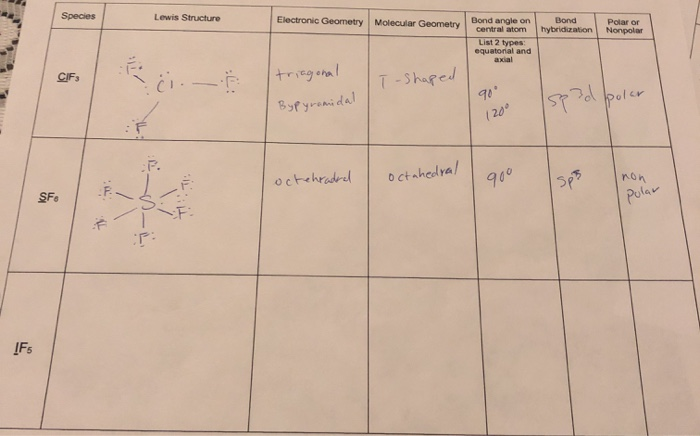Species Lewis Structure Electronic Geometry Molecular Geometry Bond hybridization Polar or Nonpolar Bond angle on central atom List 2 types equatorial and axial CIFs Eci. - T-shaped I triagonal Bypyramidal 90 120° polar 1593 octehradrel octahedral 900 F Isps non SF polar IFs MOLECULAR BONDING AND STRUCTURE ns: For each chemical species, draw the most stable Lewis structure and predict its molecular and electronic geometry about the underlined central atom using VSEPR and Orbital Hybridization theory, Predict the bond angle...

• ### Question 10 1 pts Consider the Lewis structure of IF 2 , what is the bond...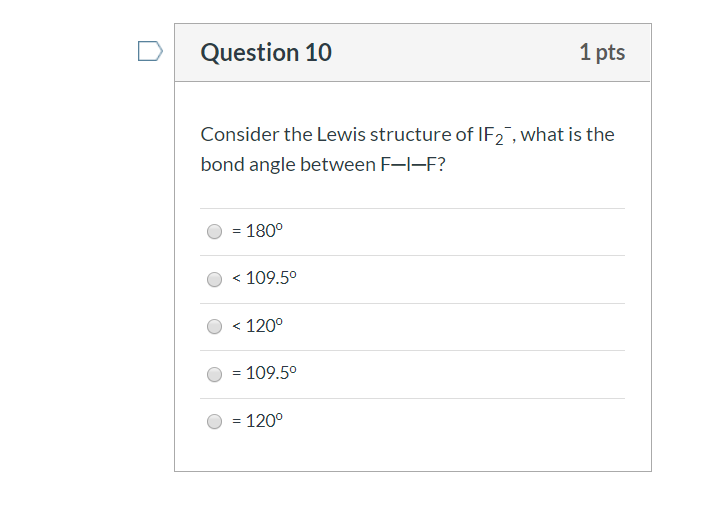Question 10 1 pts Consider the Lewis structure of IF 2 , what is the bond angle between F-I-F? = 180° < 109.5° O < 120° = 109.50 O = 120°

• ### Question 4 2.5 pts Give the molecular geometry and number of electron groups for BrF5. square...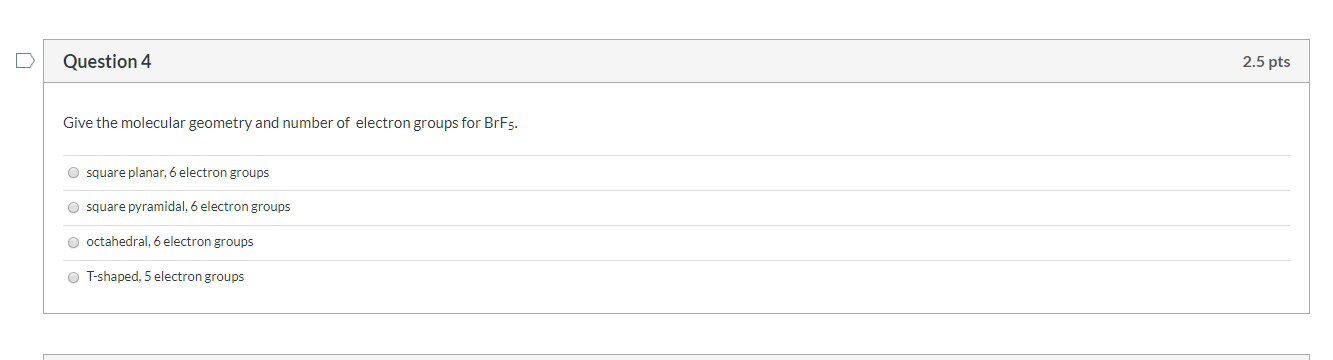Question 4 2.5 pts Give the molecular geometry and number of electron groups for BrF5. square planar, 6 electron groups square pyramidal, 6 electron groups octahedral, 6 electron groups T-shaped, 5 electron groups

• ### SCHLOSSASALARI na Due tune < Assignment Score: 66.7% Resources Question 1 of 6 > Identify the...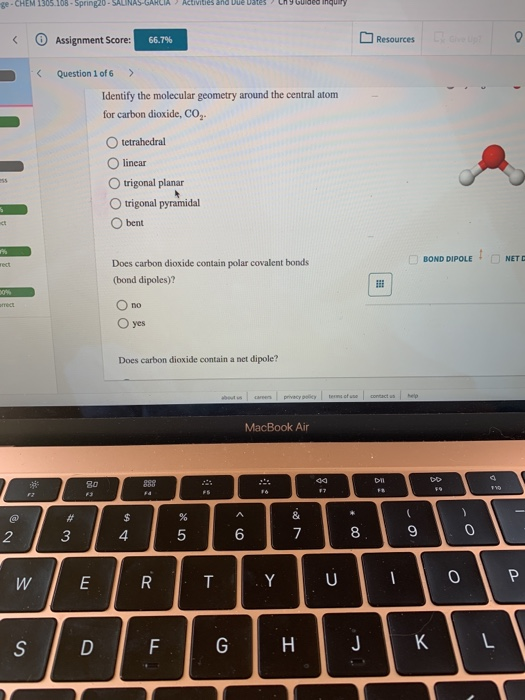SCHLOSSASALARI na Due tune < Assignment Score: 66.7% Resources Question 1 of 6 > Identify the molecular geometry around the central atom for carbon dioxide, CO, tetrahedral linear trigonal planar O trigonal pyramidal O bent Does carbon dioxide contain polar covalent bonds (bond dipoles)? BOND DIPOLE! NETE Ono O yes Does carbon dioxide contain a net dipole? MacBook Air W ΕΙ

• ### Question 8 What is the approximate bond angle around Silicon in SiF4? Choose the best response....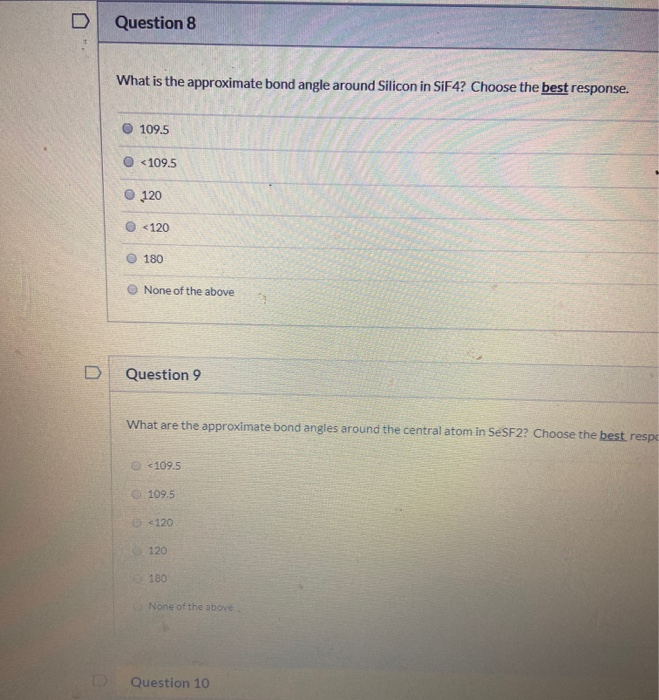Question 8 What is the approximate bond angle around Silicon in SiF4? Choose the best response. 109.5 <109.5 120 <120 180 None of the above Question 9 What are the approximate bond angles around the central atom in SeSF22 Choose the best respo <109.5 109.5 6 <120 120 180 None of the above Question 10

• ### please please quickk helpp Question 20 1.5 pts What is the molecular geometry around a central...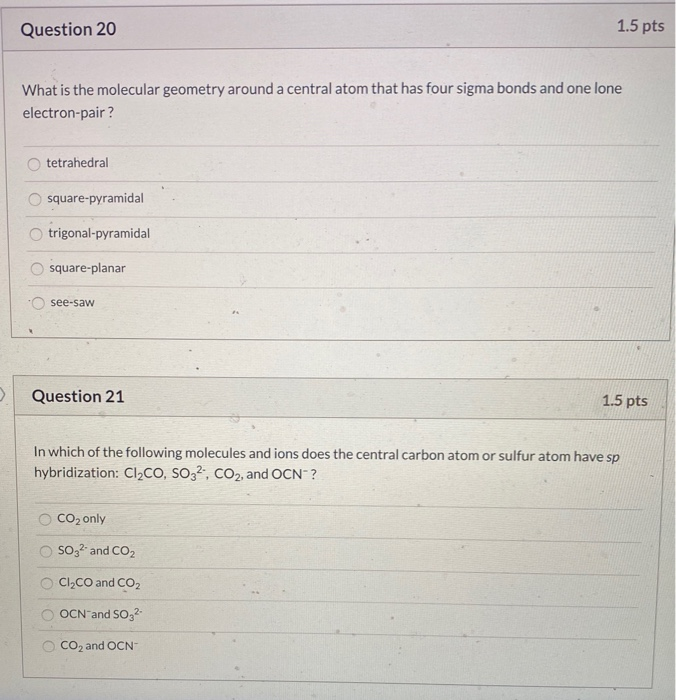please please quickk helpp Question 20 1.5 pts What is the molecular geometry around a central atom that has four sigma bonds and one lone electron-pair? tetrahedral square-pyramidal trigonal-pyramidal O square-planar o see-saw Question 21 1.5 pts In which of the following molecules and ions does the central carbon atom or sulfur atom have sp hybridization: Cl,CO, SO32, CO2, and OCN-? CO2 only SO32- and CO2 CI,CO and CO2 OCN and S032 CO2 and OCN 1.5 pts Question 19 Which...

• ### Normal text Arial 2. 3 7- The molecule of NH, is a) tetrahedral with bond angle...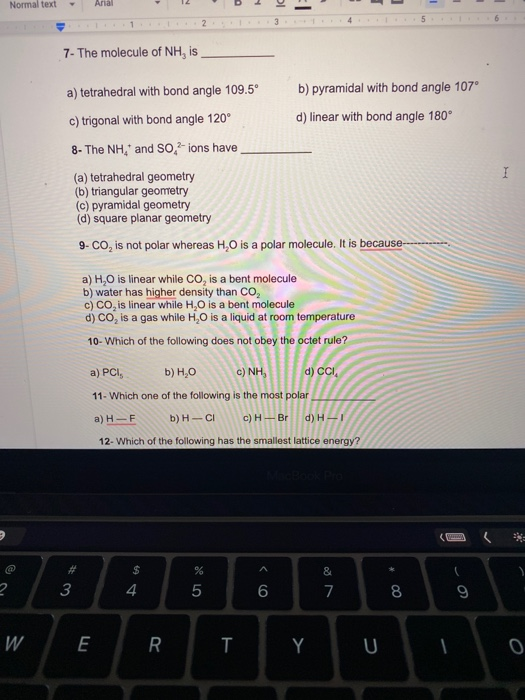Normal text Arial 2. 3 7- The molecule of NH, is a) tetrahedral with bond angle 109.5° b) pyramidal with bond angle 107° c) trigonal with bond angle 120° d) linear with bond angle 180° 8- The NH, and So, -ions have (a) tetrahedral geometry (b) triangular geometry c) pyramidal geometry (d) square planar geometry 9-CO, is not polar whereas H,0 is a polar molecule. It is because I a) H.O is linear while Co, is a bent molecule b)...